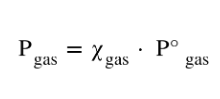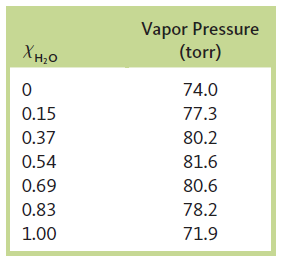# Problem: The vapor pressures of several solutions of water–propanol (CH3CH2CH2OH) were determined at various compositions, with the following data collected at 45°C:a. Are solutions of water and propanol ideal? Explain.

###### FREE Expert Solution
• An Ideal Solution are those which follow the Raoult's Law in all range of its concentration where Ptheory = Pobserved
• Raoult's Law states that the partial pressure of each component of an ideal solution of liquids is equal to the vapour pressure of the pure component multiplied by its mole fraction in the solution:99% (498 ratings)###### Problem Details

The vapor pressures of several solutions of water–propanol (CH3CH2CH2OH) were determined at various compositions, with the following data collected at 45°C:a. Are solutions of water and propanol ideal? Explain.